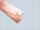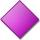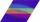# Acreage

Plot has a diamond shape, its side is 25.6 m long and the distance of the opposite sides is 22.2 meters. Calculate its acreage.

Result

S =  568.32 m2

#### Solution:Leave us a comment of example and its solution (i.e. if it is still somewhat unclear...):Be the first to comment!## Next similar examples:

1. StripFrom 5.9 cm wide strip should be cut rhombus with area 28 cm2. How long will be its side?
2. CableCable consists of 8 strands, each strand consists of 12 wires with diameter d = 0.5 mm. Calculate the cross-section of the cable.
3. Rhombus 4Circumference of the rhombus is 44 cm, its height is 89 mm long. Calculate its content area.
4. Area of ditchHow great content area will have a section of trapezoidal ditch with a width of 1.6 meters above and below 0.57 meters? The depth of the ditch is 2.08 meters.
5. RhombusPQRS is a rhombus. Given that PQ=3 cm & height of rhombus is given 2 cm. Calculate its area.
6. Rhombus HPCalculate area of the rhombus with height 24 dm and perimeter 12 dm.
7. Trapezium 2Trapezium has an area of 24 square cms. How many different trapeziums can be formed ?
8. 22/7 circleCalculate approximately area of a circle with radius 20 cm. When calculating π use 22/7.
9. AnnulusThe radius of the larger circle is 8cm, the radius of smaller is 5cm. Calculate the contents of the annulus.
10. DiagonalCan a rhombus have the same length diagonal and side?
11. Obtuse angleWhich obtuse angle is creating clocks at 17:00?
12. Simple equationSolve for x: 3(x + 2) = x - 18
13. IronIron ore contains 57% iron. How much ore is needed to produce 20 tons of iron?
14. ClassIn 7.C clss are 10 girls and 20 boys. Yesterday was missing 20% of girls and 50% boys. What percentage of students missing?
15. AverageThe arithmetic mean of the two numbers is 71.7. One number is 5. Calculate the second number.Combine like terms 4c+c-7cHow many seeds germinated from 1000 pcs, when 23% no emergence?